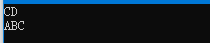2021-11-07 00:50

Query数据结构与算法问题

We have a set of strings (set A) and a set of queries (set B). Each query is to check whether a string exists in set A or not. If yes, output this string.

void query(string A[], int n, string B[], int m);

Here, n is the number of strings in set A, m is the number of strings in set B. 1<=n,m<=500,000.
Submit the function only.

No input.

Output the strings in set B which exist in set A. The output should follow the original order of the strings in set B.

For example,
A=“ABC”, A=“CD”, A=“D”
B = “A”, B =“CD”, B=“BC”, B=“ABC”,
then you should output:
CD
ABC

• 写回答
• 好问题 提建议
• 追加酬金
• 关注问题
• 收藏
• 邀请回答

2条回答默认 最新

•广大菜鸟 2021-11-07 02:34
已采纳
//利用键值对，查询比较快，时间复杂度可以达到O(n)
#include<iostream>
#include<string>
#include<map>
using namespace std;
void query(string A[], int n, string B[], int m) {
map<string, int>num_map;
for (int i = 0; i < n;i++) {
pair<string, int>value(A[i], 1);
num_map.insert(value);
}
for (int i = 0; i < m; i++) {
map<string, int>::iterator it = num_map.find(B[i]);
if (it != num_map.end()) {
cout << B[i] << endl;
}
}
}
int main() {
string A[] = { "ABC", "CD","D" };
string B[] = { "A","CD","BC","ABC" };
query(A, 3, B, 4);
}评论
解决 无用
打赏 举报